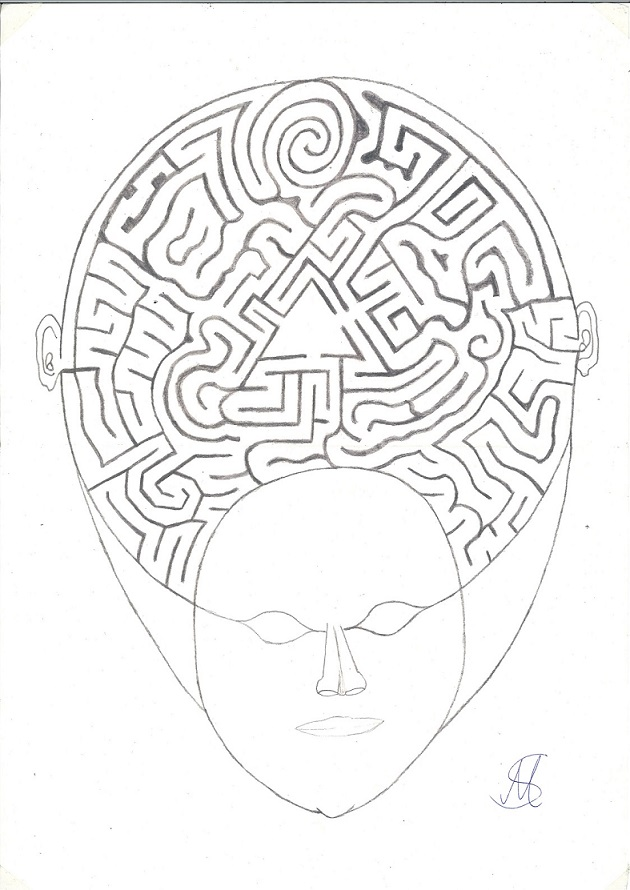# Worldstate.de

Welcome to worldstate.de or worldstate.org an idea-compilation, it should give an impuls to inventors, politicians and if nothing works out I hope someone has a follow-up idea.

## Worldstate, Estado Mundial, Etat mondial, Weltstaat, 世界状态, विश्व राज्य, мировое государство, العالم , 世界国家, negara dunia, عالمی ریاست, estado ng mundo, বিশ্ব রাষ্ট্র, thế giới, dünya devleti, รัฐโลก, stan światowy, stato mondiale, 세계 상태, Jimbo la Dunia# English

I present my project called „Worldstate“. In an (electronic) money-system that is not correspondant to economic progress the government creates money to pay companies for collective performance, which they have to proove. No infaltion. With seperation in theoretically unlimited government-money to afford all necessary infrastructure especially in 3rd world, renewable energy(faster than E.U.-Plan 2050) or space populating and civilian money which includes 5.000\$-10.000\$ average salary monthly, unemployment benefits 2.000\$, health insurance, pension/retirement money... (social system of Austria). Fixed prices. This would accelerate development, give us the time to controll environmental problems and minimize poverty and crime. The states would stay regulation districts and democracy the political system. World parliament. Then we would all work together and be limited only by the resources; resource-management! No pressure to succeed. Anyone could build up something with 20 years of hard work.

Can these rights be sued through human rights, for example the right to fair payment, the right to pollution reduction/alternative fuel, the right to the best system for fast development, the right to freedom from inflation and wealth through actual economic growth; fraud for wealth, right to fairness for the 3rd world, right to populate space; Contradiction: more money through economic growth but inflation destroys that, capping-principle; doesn't inflation come about, among other things, through comparison with other currencies and economies? The evolution of the system has developed like this, but don't we have more options with another system...all the projects that can't be funded now but would be necessary. Doesn't this monetary and economic system force us; let's dominate it!

Simple but good.

Is the debtsystem of countries better?! 100 year old debts. Not any country can pay that back, only paying the interest rate of the debt!

The countries with the best economy got the most debt!? Debt-/creditworthiness-justice.

The quality of social benefits should not depend on tax revenue.

I want to develop 3rd world to 1st world-level and realize projects simoultaniously without people from rich countries spending their money for it. No national economy can afford these projects.

I want to avoid cold war 2.0.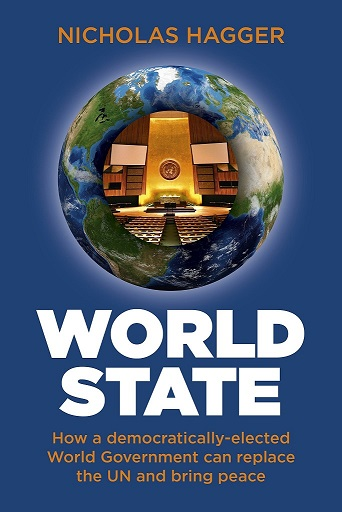book

## Space Populating

The space Populating project is a system of electromagnetic accelerators which start in the moon; like C.E.R.N. but bigger and straight to shoot us at high velocity into universe.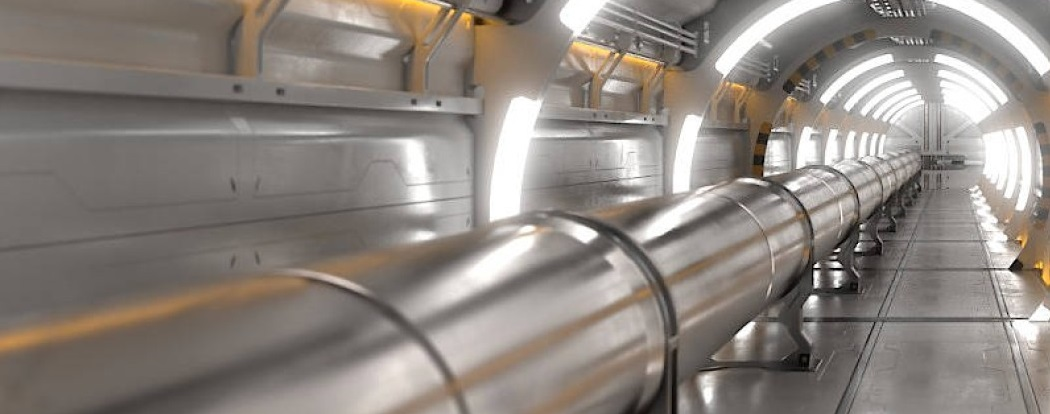electromagnetic accelerator like CERN but bigger and straight for spaceships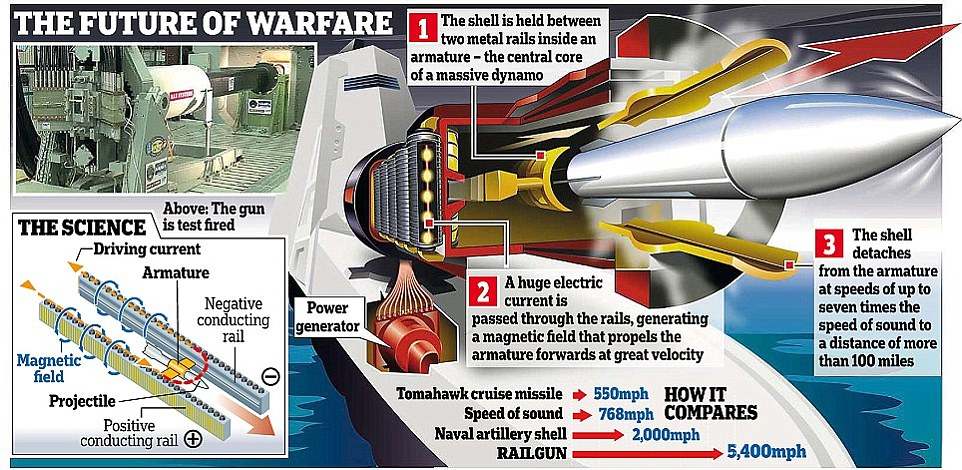Electromagnetic Railgun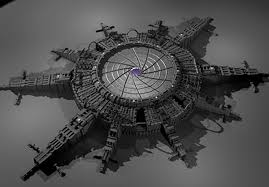Moon-Gate and Station

An electromagnetic railgun can accelerate a projectile in 5 [meters] to approximatly 10000 [km/h] divided by 3.6 = 2780 [m/s].

v^2 = u^2 + 2as

where a is the acceleration, v is the final velocity, u is the initial velocity, and s is displacement. u = 0

a = v^2 / (2s)

a = 2780^2 [m/s] / (2 * 5 [m])

a ≈ 772 840 m/s^2

Now, we can use the same acceleration to calculate the final velocity of the spaceship on the moon. Since we're given the distance traveled 3 000 000 [m] (diameter moon), we can use the following equation:

v^2 = u^2 + 2as

We're given that the distance traveled is 3,000,000 meters, so:

v = √(2as)

v = √(2 * 772 840 * 3 000 000)

v ≈ 2 153 379 m/s = 7 752 163 [km/h]

Or with the Helical-Engine.

## Or scientist discovered a warp-bubble coincidentally!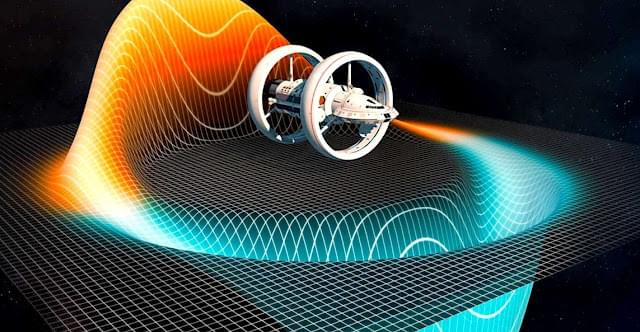Alcubierre-Drive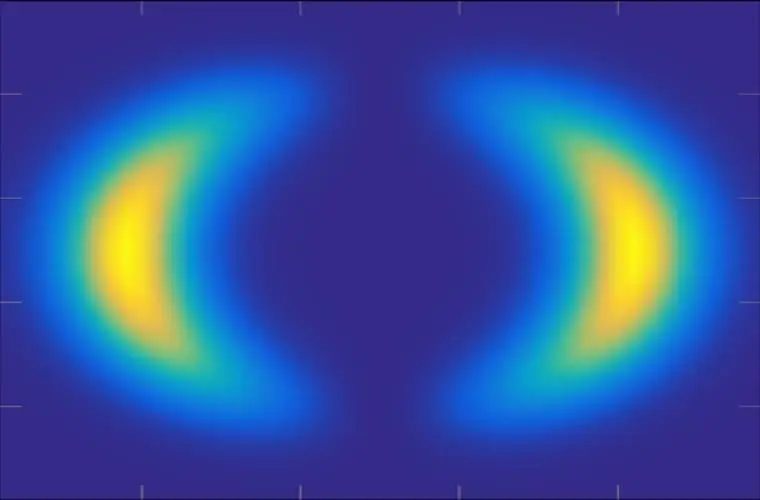Warp-Bubble

## Quantumfield-Fluctuation-Turbine (patent application)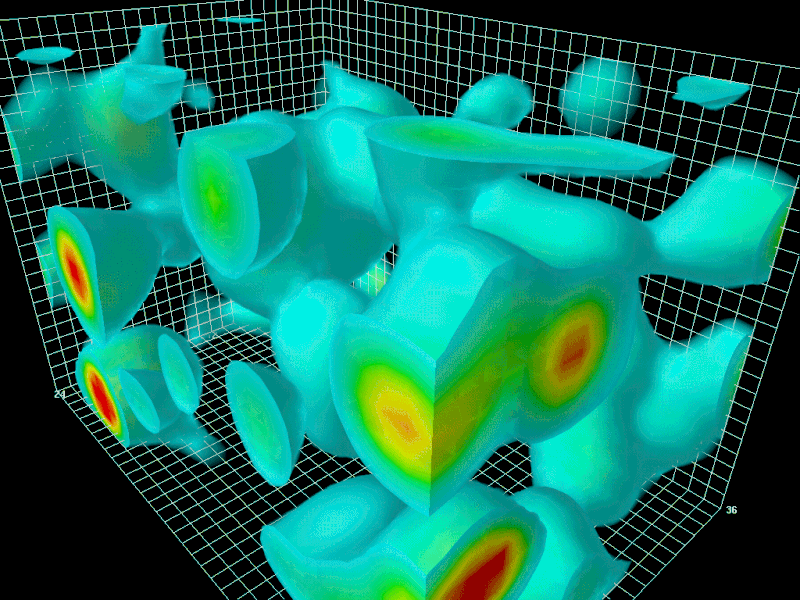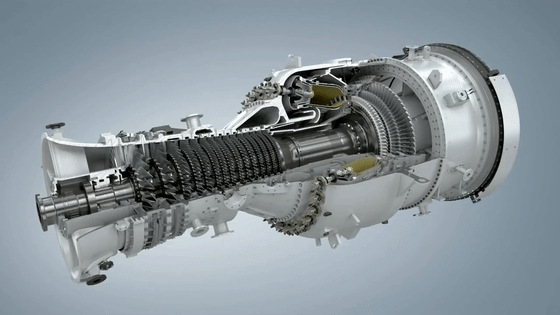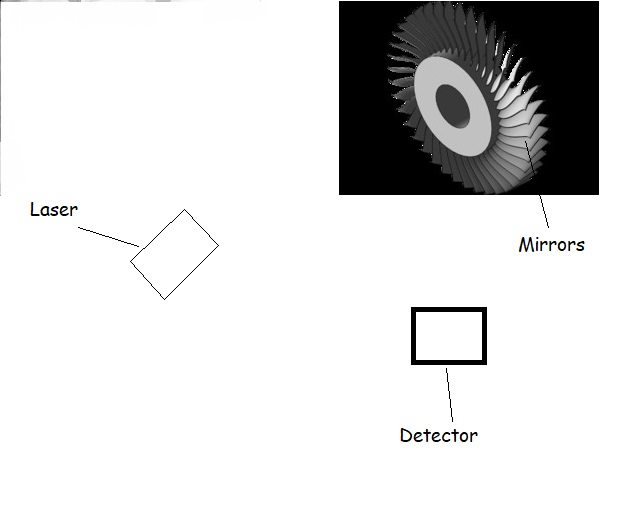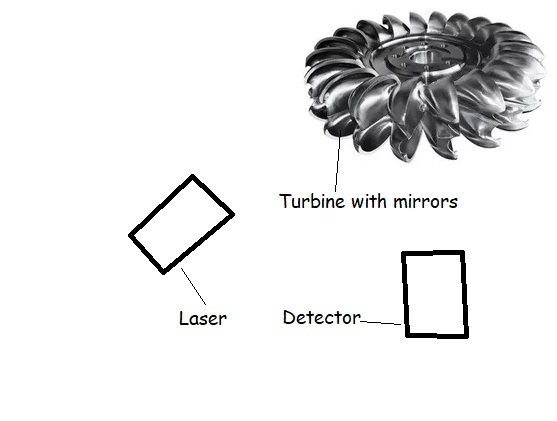I use 200kW lasers, detectors and silicium-mirrors which are static and completely reflecting instead of the turbine-leaves and it will rotate because of the quantum-fluctuations, quantum noise and the radiation pressure.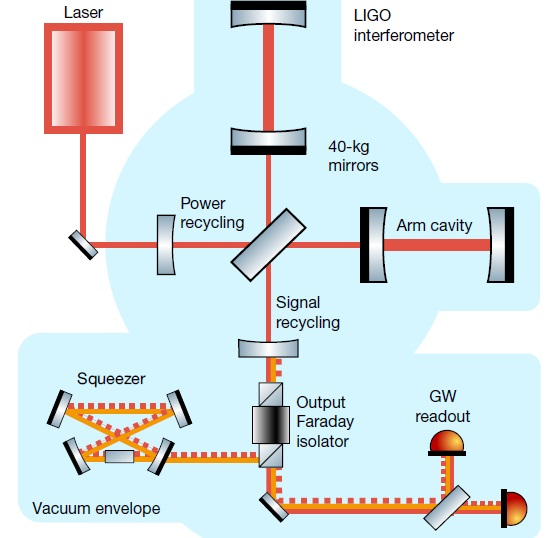p_st or light pressure is the pressure exerted on a surface by absorbed, emitted or reflected electromagnetic radiation. For absorption and emission, the radiation pressure is equal to the intensity I of the wave divided by the speed of light c: p_st = I/c with the units p_st = N/m² = J/m³ = Pa (Pascal); In the case of complete reflection, the radiation pressure is twice as great as in the case of complete absorption. A photon of frequency ν transports the energy: E = h * ν (see Photoelectric Effect) with the Planck constant h.

Because of the energy-momentum relation E = (p²*c² + m²*c4)^1/2 for the photon with a mass m = 0 follows a momentum p with the magnitude: | p | = h*ν /c

Wave model: The pressure exerted on a surface by a radiation field in a vacuum can be expressed by the Maxwell's stress tensor ( T i j ). With an absorbing surface Normal vector n is the radiation pressure given by: p_st*nj = ∑ Tij* ni The components of Maxwell's stress tensor can be derived from the electrical Calculate the field strength E and the magnetic flux density B:

Tij = (1/ 2)*( ε_0*E² + B²/ μ_0 )*δij − ε_0*Ei*Ej − Bi*Bj/ μ_0

where δij is the Kronecker delta, ε_0 the electric field constant and μ_0 the magnetic field constant is.

Quantum Noise

arises from the quantization of electromagnetic radiation and has a spectral power density of hf. For wavelengths ≤ 10 μm, thermal noise predominates. Optical receivers in the visible range therefore require significantly higher signal power than in the range of electronic frequencies. This occurs when atoms or subatomic particles (electrons, photons or even smaller particles) are involved in an energetic level transition (“quantum jump”). Corresponding measured values ​​(and thus calculation results) are subject to a Probability distribution and are mathematically-probabilistically correct, in the computational practice but often unsatisfactory to unusable.

There is as yet no formulation of the force exerted by quantum fluctuations

## ManualHandFanCasimirEffect (patent application)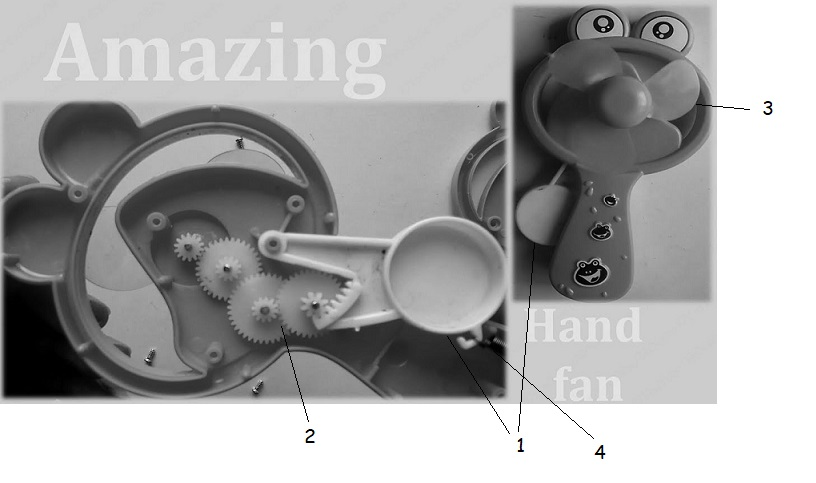Mechanics of a manual hand fan (3) with return spring (4), drive lever (1) and gear power transmission (2)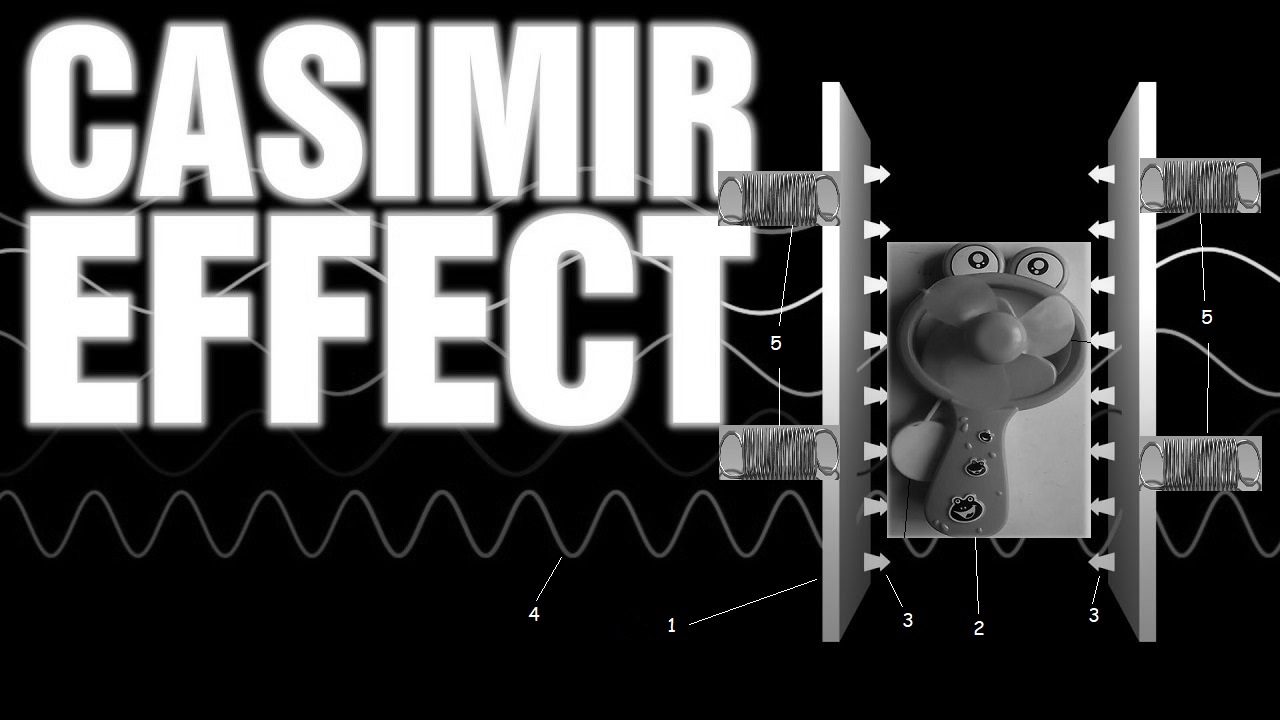consisting of Casimir effect panels (1), manual hand fan (2) with Return springs (5), Casimir force (3) and quantum fields/fluctuations in different wavelengths (4)

The manual hand fan generates rotational energy by repeatedly applying pressure to a lever using the Casimir effect, causes an air flow through a fan through a gear mechanism and is returned to the starting position of the lever by a spring or rubber ring.

## Seafood-Farm

A Seafoodfarm with the surface of state New York could feed the whole world.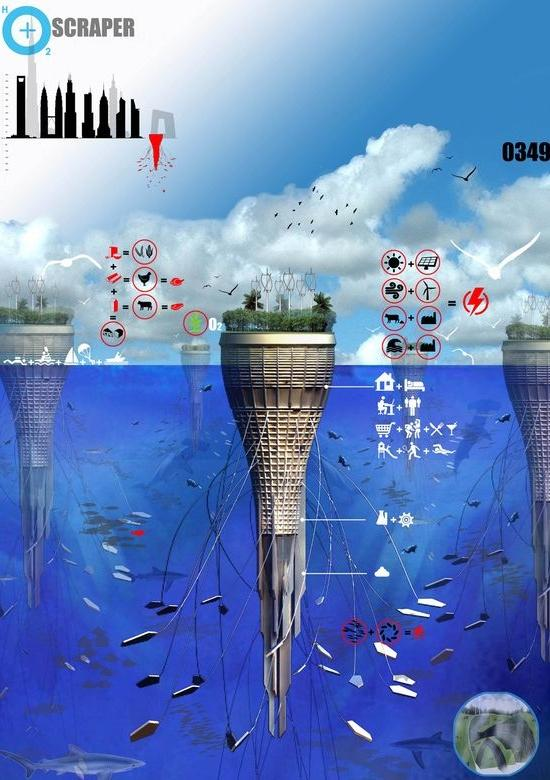Ocean Bottom Scraper, gigantic seafood-farm

## carbonengineering.com

The company invented a process to produce a granulate from the carbon dioxide in the air and use it as fuel.

One facility absorbs as much CO2 as 40 million trees; every gasstation gets a facility to produce the fuel on site.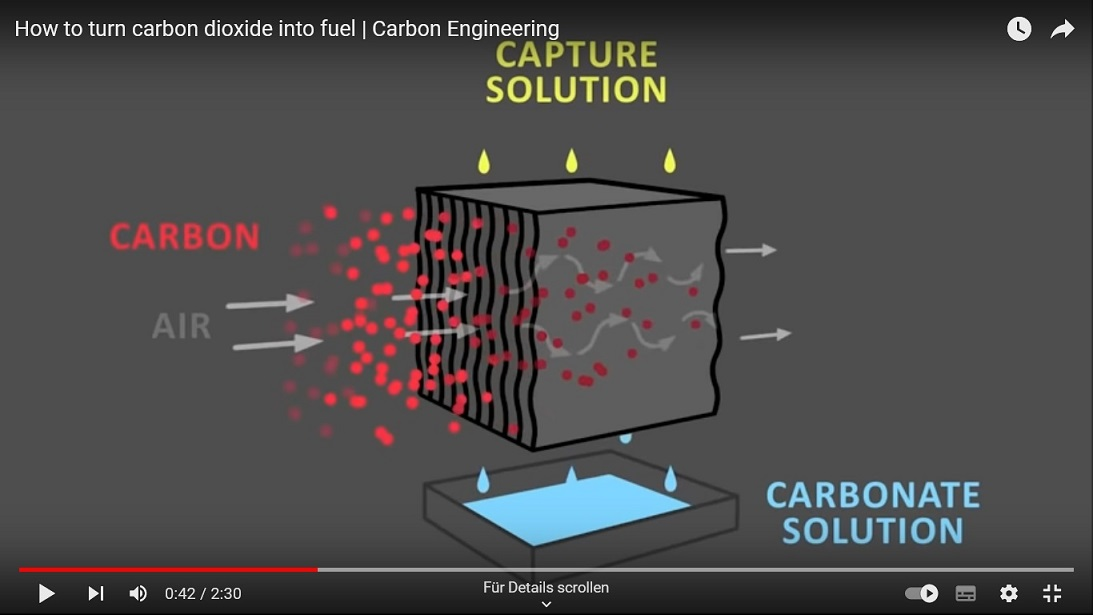Carbon-Engineering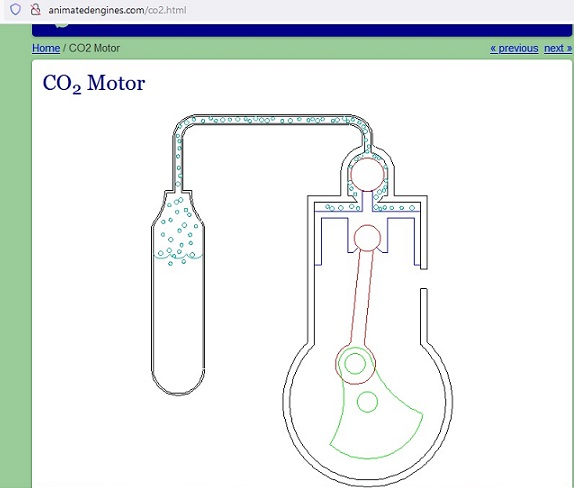CO2-Engine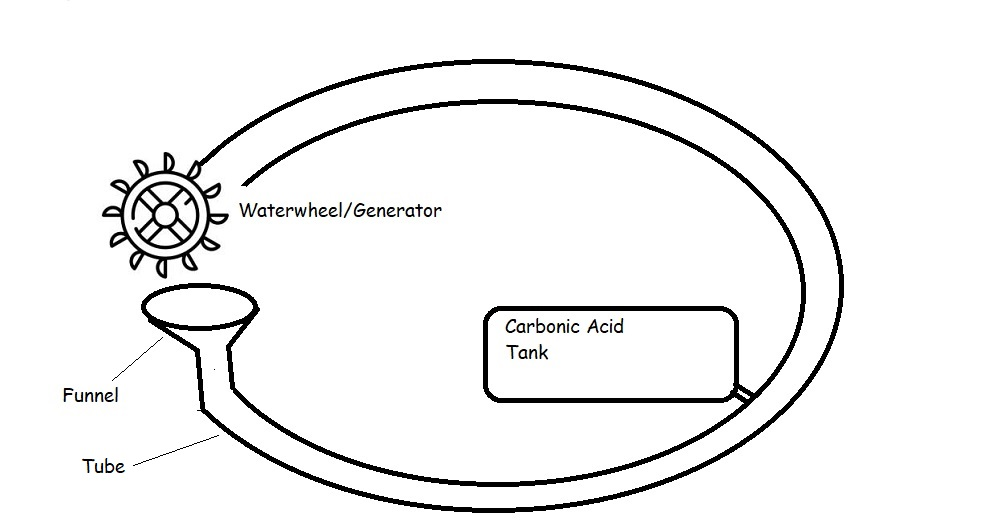Robert Boyle's Flask works with beer until the carbonic acid disappears. The video on youtube.com is called: “Boyle’s flask works now”. By adding a water wheel as a generator and a sparger for infiltrating carbon dioxide or gas bubbles or a carbonic acid tank the cycle is maintained to win energy.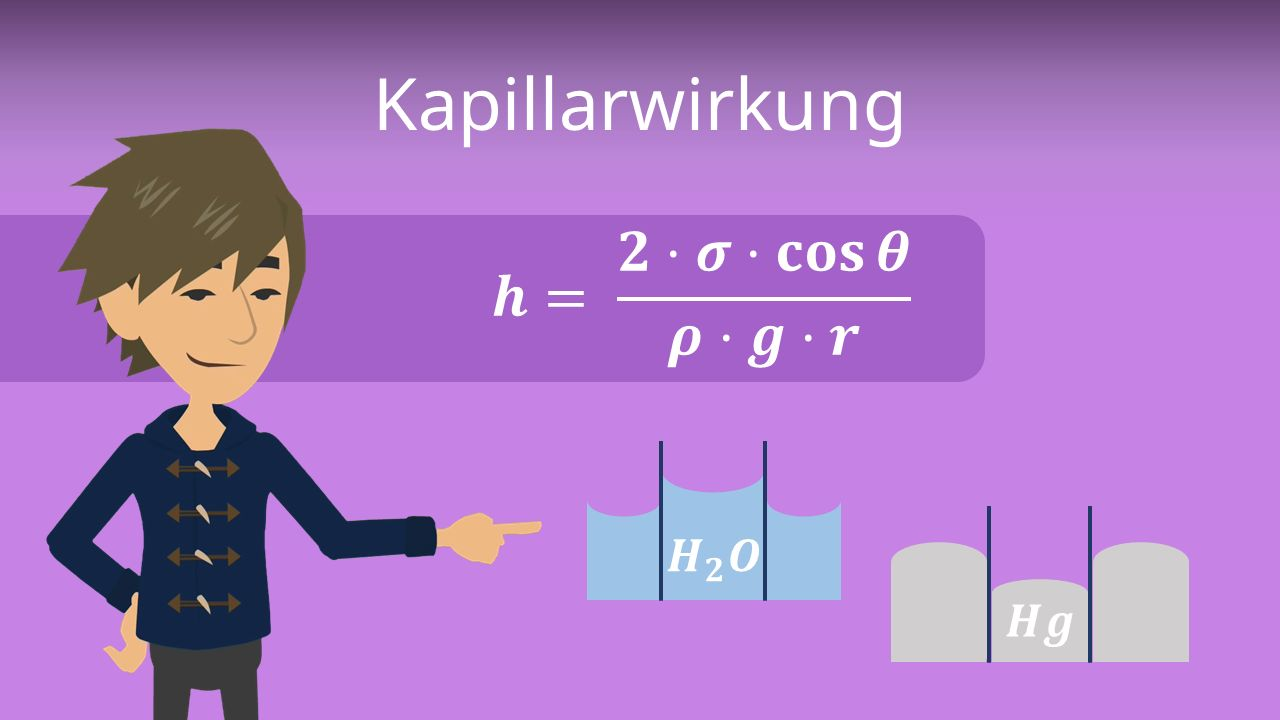CapillaryFormulaClimbingHight

h = climbing height; σ = surface tension; θ = contact angle; ϱ = density; g = acceleration of gravity; r = radius.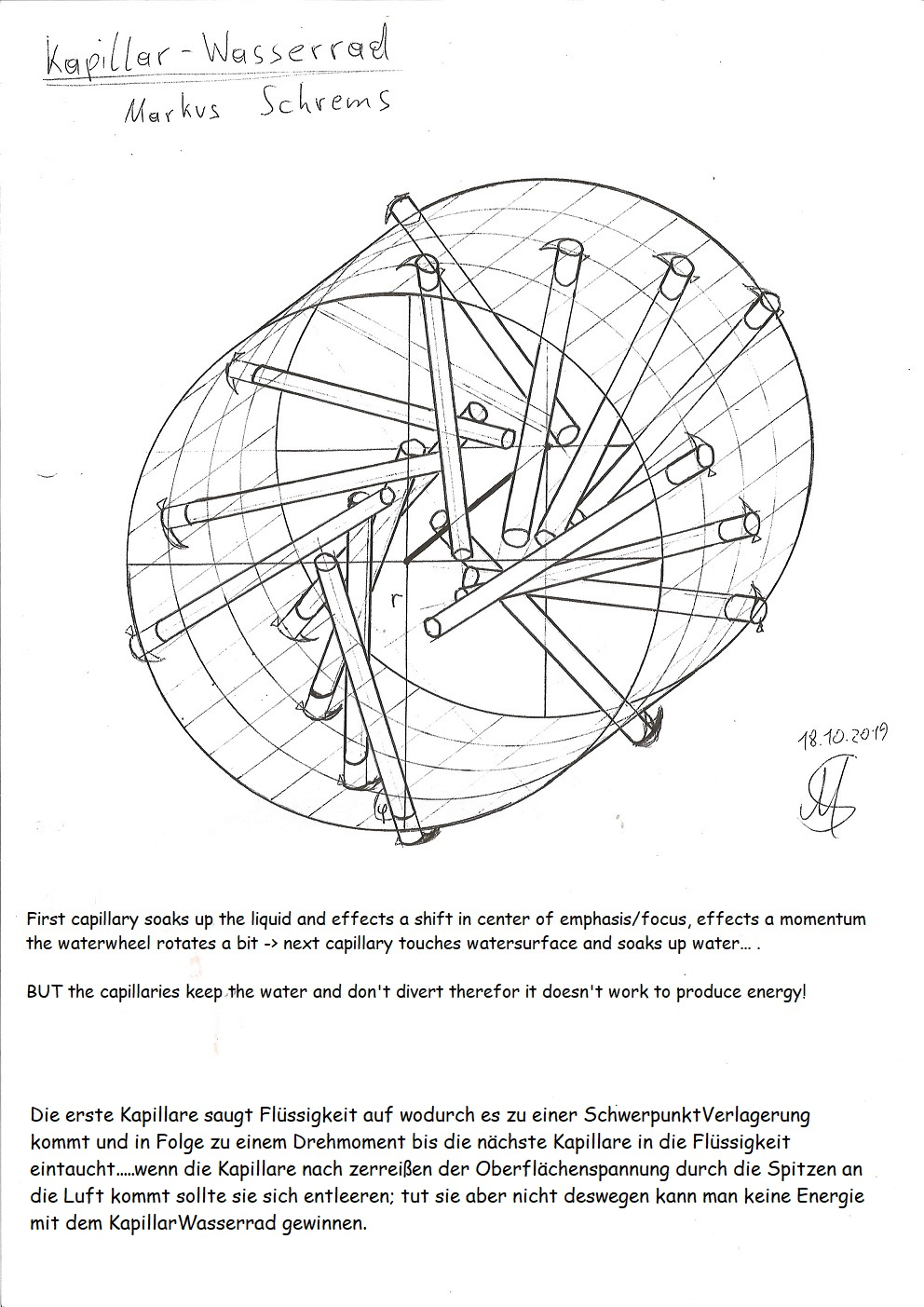Capillary Waterwheel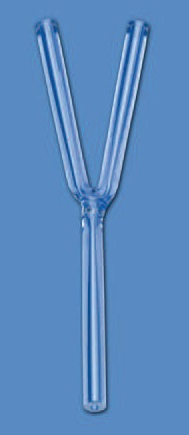YCapillary fills untill both upper ends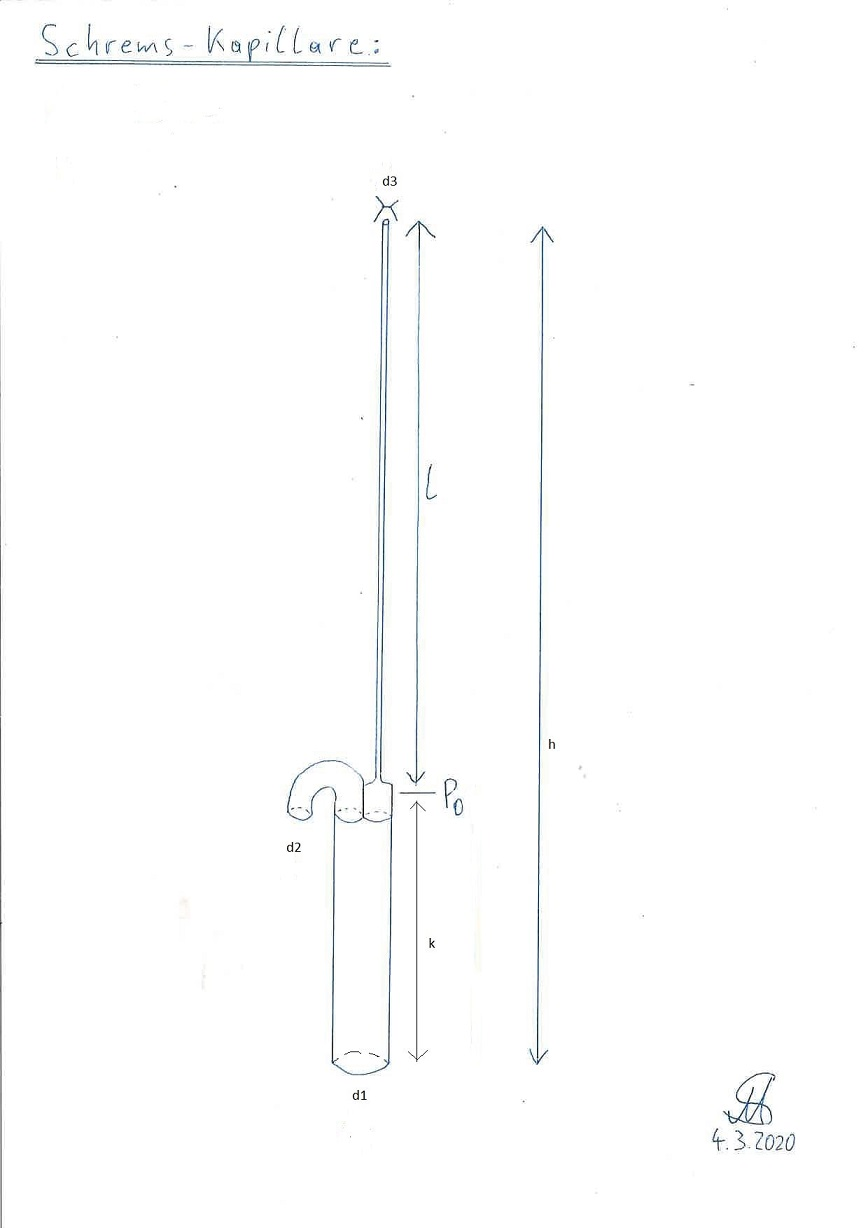Schrems-Capillary: should fill itself completely, but a prototype with measures: k = 3cm; L = 1m; d1 = 0,8mm; d2 = 0,4mm; d3 = 0,02mm didn't work. It filled itself but the pressure at d2 was too low so it didn't divert there.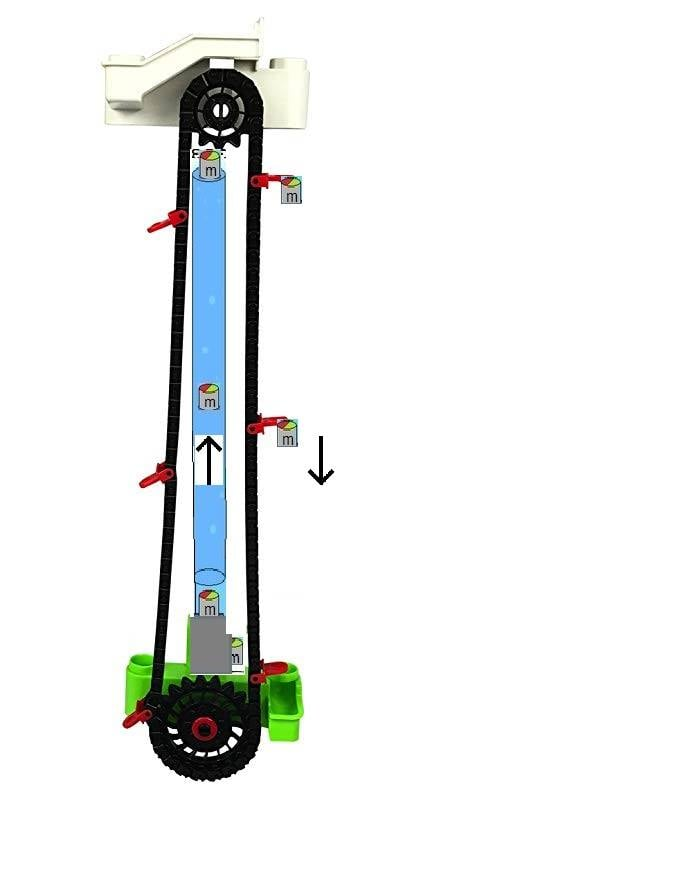UpliftLift

Masses lighter than water are infiltrated into the water column and move through it hydrostatic uplift upwards. At the upper end the masses are lifted away by the lift and mounted on the lift, they fall downwards to keep the lift moving to generate energy. The uplift lift can also be operated with oil instead of the masses and do the infiltration with a nozzle.

## Valve capillary (Patent application)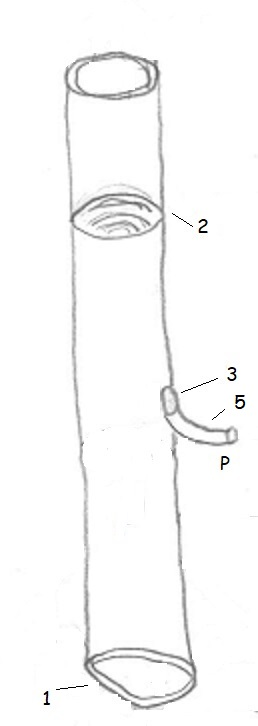The valve capillary sucks the liquid according to the capillary effect of the diameter of the Capillary-dependent rise height (2) through open flaps (1) whereby the valve (3) is closed. Then the flaps (1) close and the valve (3) (both electrically) opens to divert with gravity-pressure P the liquid at a higher level. Then the valve (3) closes, the closure flaps open and new liquid is sucked up by the capillary effect...

## WettingCapillary (patent application)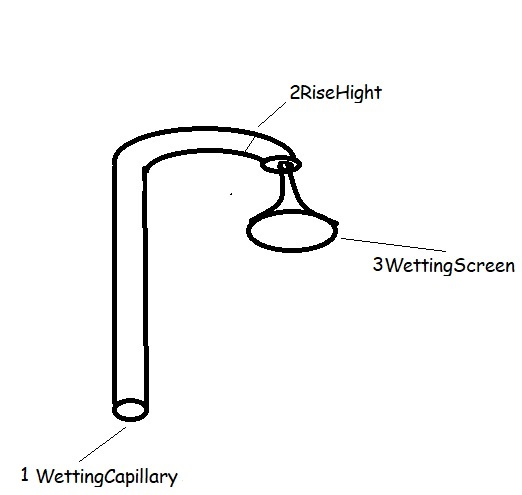The wetting capillary (1) sucks up the water up to the rise height h (2), which depends on the diameter, and is curved downwards in the upper area. The wetting screen (3) is thinner at the tip than the capillary and the contact angle is very small, which means that the liquid spills over and drips off at the bottom of the screen.

My patent applications are in the direction of perpetual motion and conscious I keep quiet about the production of energy or electricity because otherwise 100% rejection occurs. However, based on my conviction, I am of the opinion that CarnotCycle, energy conservation laws and the proof of the impossibility of a Perpetuum mobiles, i.e. efficiency less than or equal to 1 ONLY for thermodynamic machines applies like motors and there is a loophole.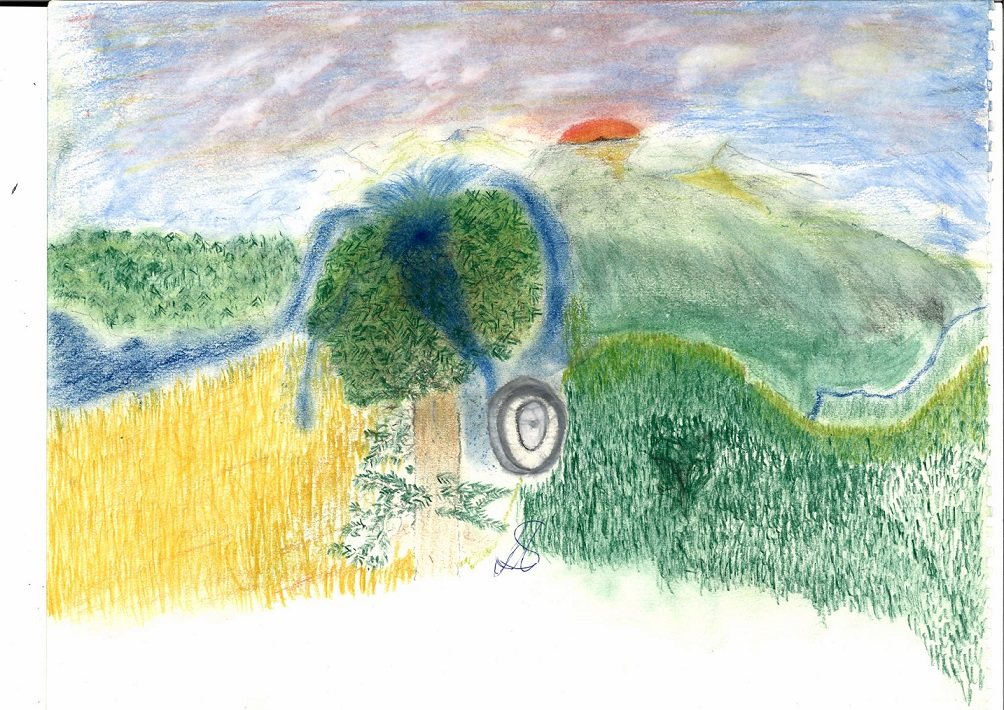SequoyahCapillaryPowerplant

## Desertification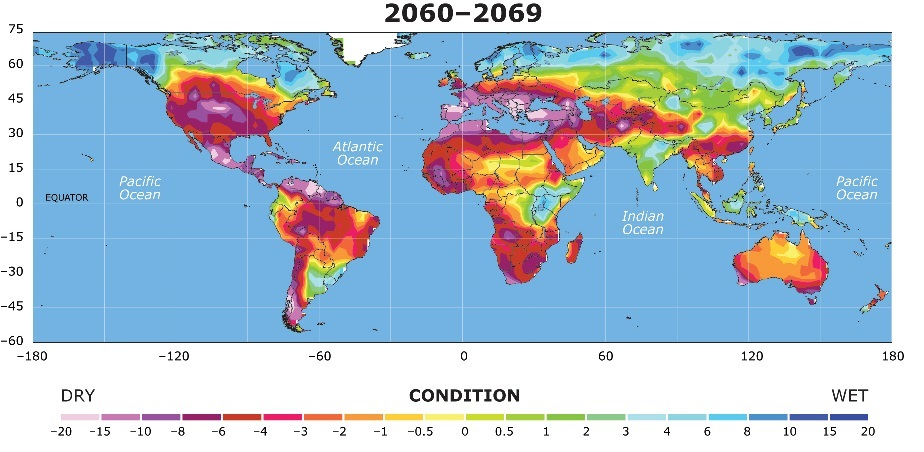Desertification

## Acceleration to high velocity

To accelerate a human at 6G to half the speed of light 29 days or 2527232 seconds of acceleration are necessary.

t = (c/2)/a = (149896229[m/s]) / 59[m/s^2] = 2527232 seconds

The total energy required to accelerate a 1000 kg spaceship to half the speed of light at 6G is approximately:

W = F * d

F = 1000 [kg] * 59.28 [m/s^2]

F = 59280 [N]

d = (1/2) * a * t^2

d = (1/2) * 59 [m/s^2] * (2527232 [s])^2

d = 2 * 10^14 [meters]

W = F * d

W = 59280 [N] * 2 * 10^14 [m]

W = 1*10^19 [joules]

To determine the amount of energy that a single Tokamak ITER reactor can produce in 29 days, we need to convert 29 days to seconds, which is 2527232 seconds. The energy produced by a single Tokamak ITER reactor with a power output of 1.5 GW in 29 days is:

energy = power * time

energy = 1.5 [GW] * 2527232 [seconds]

energy = 4 * 10^15 [joules]

W / energy = 1*10^19 [joules] / (4 * 10^15 [joules]) = 2500

Therefore, it would require approximately 2500 Tokamak ITER reactors, each producing 1.5 GW of power, running continuously for 29 days to generate the energy required to accelerate a 1000 kg spaceship to half the speed of light at 6G.

or

E_kinetic = (1/2)*m*v²

E_kinetic = (1/2) * 1000 [kg] * (149896229 [m/s])^2

E_kinetic = 1 * 10^19 [joules]

...

## Levitation (patent application)

Why jumps a Popcorn-Pot?

ImpulsRoof > ImpulsFloor + AccelerationRecoil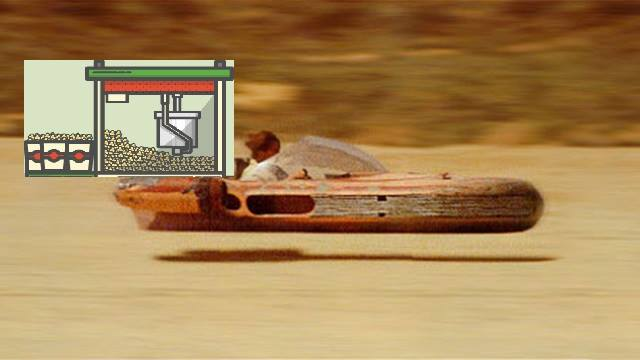PopcornMashineLandspeeder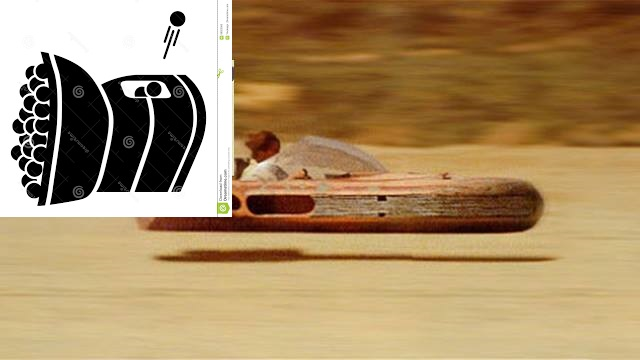TennisballMashineLandspeeder

Shoot iron balls diagonally up to the ceiling of the chamber so that the falling balls can be reused.

I use electromagnetic acceleration with the balls in the center of a ring-shaped electromagnetic accelerator, that is, in the center of a hollow cylinder or a coil to have little or no recoil.

Never with a railgun, the recoil is too great.

Conservation of momentum:

m_1*v_1 + m_2*v_2 = m_1*v_1' + m_2*v_2'

Q is flow (Q = V/t; in m³/s)

ρ is the density (kg/m³)

V is the volume (in m³)

m is the mass (in kg)

v is velocity (in m/s))

g is acceleration due to gravity (9.8 m/s2)

t is Time (in seconds)

Impuls: p = m*v

Force: F = m*dv/dt = m*a

But the formula must be something like:

F_g = (Δv*m)/dt = (Δv*V*ρ)/dt = Δv*Q*ρ = Δv*Q*γ/g = Δv*ṁ

Δv is velocety-difference between impulse roof and impulse floor

γ specific weight in N/m³ = kg/(m²s²)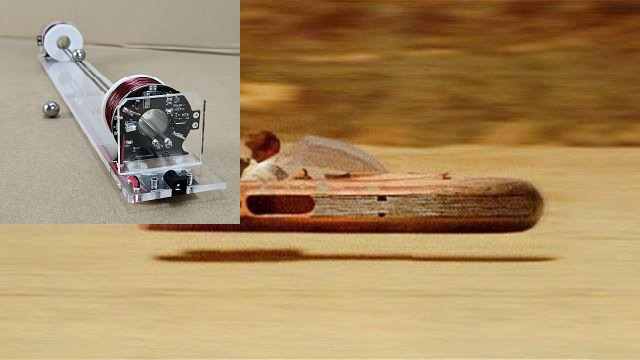BallElectromagneticAccelerationLandspeeder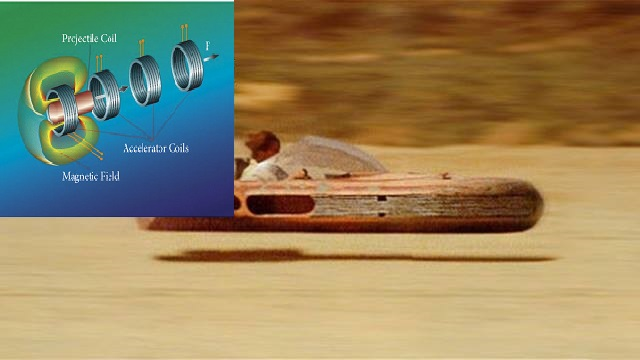ElectromagneticAcceleratorLandspeeder

A motor generates the energy for the electromagnetic acceleration. Several such iron-ball-electromagnetic-accelerator-chambers are combined to make a load such as a skateboard or a car levitate or fly.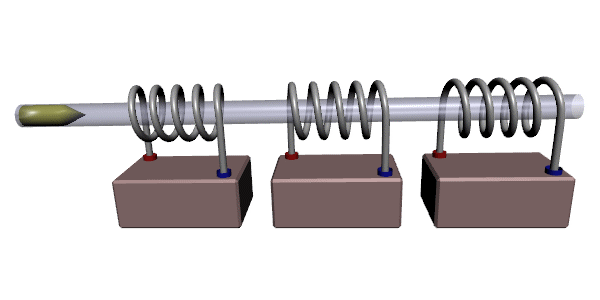coil gun

v_end = ((2*V* μ_0*χ_m*n²*I²)/m)^1/2

m is mass of the projectile

V is volume projectile

χ_m is magnetic susceptibility

μ_0 is vacuum permeability 4π × 10−7 V*s/(A*m)

I is current in amperes

n is the number of coil turns per unit length of coil obtained by dividing the Total turns of the coil can be determined by the total length of the coil in meters

Force Coilgun:

F = (0.5 * B^2 * A * n * l * I^2) / d

B is the magnetic field strength inside the coilgun (Tesla)

A is the cross-sectional area of the coil (square meters)

n is the number of turns in the coil

l is the length of the coil (meters)

I is the current passing through the coil (Amperes)

d is the distance over which the force is applied (meters)